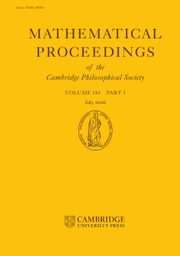Home
Hostname: page-component-544b6db54f-2p87r Total loading time: 0.193 Render date: 2021-10-20T15:01:38.558Z Has data issue: true Feature Flags: { "shouldUseShareProductTool": true, "shouldUseHypothesis": true, "isUnsiloEnabled": true, "metricsAbstractViews": false, "figures": true, "newCiteModal": false, "newCitedByModal": true, "newEcommerce": true, "newUsageEvents": true }Mathematical Proceedings of the Cambridge Philosophical Society

# Singular moduli and the distribution of partition ranks modulo 2

Published online by Cambridge University Press:  18 December 2015

## Abstract

In this paper, we prove an asymptotic formula with a power saving error term for traces of weight zero weakly holomorphic modular forms of level N along Galois orbits of Heegner points on the modular curve X0(N). We use this result to study the distribution of partition ranks modulo 2. In particular, we give an asymptotic formula with a power saving error term for the number of partitions of a positive integer n with even (respectively, odd) rank. We use these results to deduce a strong quantitative form of equidistribution of partition ranks modulo 2.

Type
Research Article
Information

## Access options

Get access to the full version of this content by using one of the access options below. (Log in options will check for institutional or personal access. Content may require purchase if you do not have access.)

## References

[Al]Alfes, C.Formulas for the coefficients of half-integral weight harmonic Maass forms. Math. Z. 277 (2014), 769795.CrossRefGoogle Scholar
[An]Andrews, G. E.On the theorems of Watson and Dragonette for Ramanujan's mock theta functions. Amer. J. Math. 88 (1966), 454490.CrossRefGoogle Scholar
[BH]Blomer, V. and Harcos, G.Hybrid bounds for twisted L-functions. J. Reine Angew. Math. 621 (2008), 5379.Google Scholar
[BO]Bringmann, K. and Ono, K.The f(q) mock theta function conjecture and partition ranks. Invent. Math. 165 (2006), 243266.CrossRefGoogle Scholar
[BJO]Bruinier, J. H., Jenkins, P. and Ono, K.Hilbert class polynomials and traces of singular moduli. Math. Ann. 334 (2006), 373393.CrossRefGoogle Scholar
[BrO]Bruinier, J. H. and Ono, K.Algebraic formulas for the coefficients of half-integral weight harmonic weak Maass forms. Adv. Math. 246 (2013), 198219.CrossRefGoogle Scholar
[C]Cox, D. A.Primes of the Form x2 + ny2. Fermat, Class Field Theory, and Complex Multiplication. Second edition. Pure and Applied Mathematics (Hoboken). (John Wiley and Sons, Inc., Hoboken, NJ, 2013), xviii+356 pp.CrossRefGoogle Scholar
[D]Duke, W.Modular functions and the uniform distribution of CM points. Math. Ann. 334 (2006), 241252.CrossRefGoogle Scholar
[Dy]Dyson, F.Some guesses in the theory of partitions. Eureka (Cambridge) 8 (1944), 1015.Google Scholar
[GKZ]Gross, B., Kohnen, W. and Zagier, D.Heegner points and derivatives of L–series. II. Math. Ann. 278 (1987), 497562.CrossRefGoogle Scholar
[GZ]Gross, B. and Zagier, D.Heegner points and derivatives of L–series. Invent. Math. 84 (1986), 225320.CrossRefGoogle Scholar
[HM]Harcos, G. and Michel, P.The subconvexity problem for Rankin-Selberg L-functions and equidistribution of Heegner points. II. Invent. Math. 163 (2006), 581655.CrossRefGoogle Scholar
[HR]Hardy, G. H. and Ramanujan, S.Une formule asymptotique pour le nombre des partitions de n. Comptes Rendus 2 (1917). Found in Collected Papers of Srinivasa Ramanujan (AMS Chelsea Publ., Providence, RI, 2000), 239241.Google Scholar
[HB]Heath-Brown, D. R.Hybrid bounds for Dirichlet L-functions. II. Quart. J. Math. Oxford Ser. 31 (1980), 157167.CrossRefGoogle Scholar
[HL]Hoffstein, J. and Lockhart, P.Coefficients of Maass forms and the Siegel zero. With an appendix by Dorian Goldfeld, Hoffstein and Daniel Lieman. Ann. of Math. 140 (1994), 161181.CrossRefGoogle Scholar
[I]Iwaniec, H.Introduction to the spectral theory of automorphic forms. Biblioteca de la Revista Matemática Iberoamericana (Revista Matematica Iberoamericana, Madrid, 1995). xiv+247 pp.Google Scholar
[I2]Iwaniec, H.Topics in Classical Automorphic Forms. Graduate Studies in Math. 17 (American Mathematical Society, Providence, RI, 1997) xii+259 pp.CrossRefGoogle Scholar
[IK]Iwaniec, H. and Kowalski, E.Analytic Number Theory. American Mathematical Society Colloquium Publications, 53 (American Mathematical Society, Providence, RI, 2004), xii+615 pp.CrossRefGoogle Scholar
[M]Masri, R.Fourier coefficients of harmonic weak Maass forms and the partition function. Amer. J. Math. 137 (2015), 10611097.CrossRefGoogle Scholar
[O]Ono, K.Unearthing the visions of a master: harmonic Maass forms and number theory. Current Developments in Math. 2008 (Int. Press, Somerville, MA, 2009), 347454.Google Scholar
[R]Ramanujan, S., The Lost Notebook and Other Unpublished Papers (Narosa, New Delhi, 1988).Google Scholar
[W]Waldspurger, J.-L.Sur les valeurs de certaines fonctions L automorphes en leur centre de symétrie. Composition Math. 54 (1985), 173242.Google Scholar
[Za]Zagier, D.Traces of singular moduli. Motives, Polylogarithms and Hodge theory, Part I (Irvine, CA, 1998), 211244. Int. Press Lect. Ser. 3, I (Int. Press, Somerville, MA, 2002).Google Scholar
[Za2]Zagier, D.Ramanujan's Mock Theta Functions and Their Applications (after Zwegers and Bringmann–Ono). Séminaire Bourbaki. Vol. 2007/2008. Astérisque 326 (2009), Exp. No. 986 (2010), viiviii, 143164.Google Scholar
[Zh]Zhang, S.Gross–Zagier formula for GL 2. Asian J. Math. 5 (2001), 183290.CrossRefGoogle Scholar
5
Cited by

# Send article to Kindle

Note you can select to send to either the @free.kindle.com or @kindle.com variations. ‘@free.kindle.com’ emails are free but can only be sent to your device when it is connected to wi-fi. ‘@kindle.com’ emails can be delivered even when you are not connected to wi-fi, but note that service fees apply.

Find out more about the Kindle Personal Document Service.

Singular moduli and the distribution of partition ranks modulo 2
Available formats
×

# Send article to Dropbox

To send this article to your Dropbox account, please select one or more formats and confirm that you agree to abide by our usage policies. If this is the first time you use this feature, you will be asked to authorise Cambridge Core to connect with your <service> account. Find out more about sending content to Dropbox.

Singular moduli and the distribution of partition ranks modulo 2
Available formats
×

# Send article to Google Drive

To send this article to your Google Drive account, please select one or more formats and confirm that you agree to abide by our usage policies. If this is the first time you use this feature, you will be asked to authorise Cambridge Core to connect with your <service> account. Find out more about sending content to Google Drive.

Singular moduli and the distribution of partition ranks modulo 2
Available formats
×
×